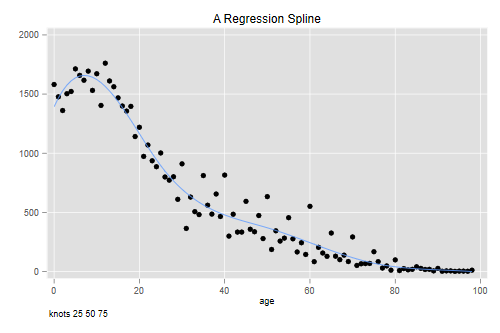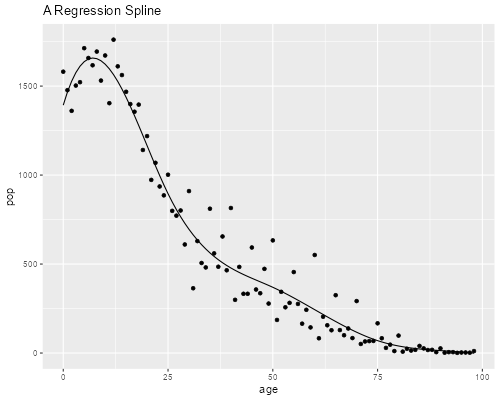Germán Rodríguez
Demographic Methods Princeton University## Smoothing: Splines

This continues our work on smoothing. Let's start by making sure we have loaded the data.

```. infile age pop using ///
>   https://grodri.github.io/datasets/cohhpop.dat, clear
```
```> co <- read.table("https://grodri.github.io/datasets/cohhpop.dat",
```

Our application will focus on regression splines, because they are the easiest ones to use, but we will mention briefly natural regression splines and smoothing splines.

### Regression Splines

A cubic spline S(x) with knots t1 … tk has linear, quadratic and cubic terms on x, and one term of the form (x - t1)3+ for each knot. The + notation instructs us to take the positive part of the argument. These terms are easy to compute and can be entered as predictors in a regression, although one should be careful about multi-collinearity when there are lots of knots.

A better solution is to use b-splines, a well-conditioned basis for splines. Stata does not have built-in b-splines, but Roger Newson has contributed a command called `bspline`.R has a function `bs()` included in the `splines` package as part of the base installation.The Stata and R implementations use somewhat different bases, but lead to the same fitted values.

Let us use spline regression to smooth the Colombian data. We will use a cubic spline with three internal knots at ages 25, 50 and 75. This spline has a total of 7 parameters. In `bspline` you need to specify the minimum and maximum as knots. You should alsospecify `p(3)` to get cubic splines (the default is linear) and seven output variables.In R the spline basis may be specified as part of the model.

```. bspline, xvar(age) knots(0 25 50 75 100) p(3) gen(_bs3k)
```

We now regress the population counts on the spline basis omitting the constant (or one of the generated variables).I skip the detailed output because we are interested in the fitted values only.

```. quietly regress pop _bs3k*, noconstant

. predict bs3k
(option xb assumed; fitted values)

. twoway (scatter pop age)(line bs3k age) , legend(off)  ///
>   note(knots 25 50 75) title(A Regression Spline)

. graph export cohhrs.png, width(500) replace
file cohhrs.png saved as PNG format
``````> library(splines)
> library(dplyr)
> library(ggplot2)
> sf <- lm(pop ~ bs(age, knots=c(25, 50, 75)), data=co)
> co <- mutate(co, smooth=fitted(sf))
> ggplot(co, aes(age, pop)) + geom_point() +
+   geom_line(aes(age, smooth)) + ggtitle("A Regression Spline")
> ggsave("cohhrsr.png", width=500/72, height=400/72, dpi=72)
```As you can see, the spline does an excellent job smoothing the data. Try using four knots at ages 20, 40, 60 and 80. The fit will look very similar. Placing the knots is an art; a common choice is to place them at given quantiles, for example the quartiles Q1, Q2 and Q3 if you want three internal knots. The number of knots is chosen to balance smoothness and goodness of fit.

### The Power Series

Just to convince yourself that there is nothing magic about b-splines, we will reproduce the results “by hand” using the power series as described in the handout

```. gen age2 = age^2

. gen age3 = age^3

. gen k25 = cond(age > 25, (age - 25)^3, 0)

. gen k50 = cond(age > 50, (age - 50)^3, 0)

. gen k75 = cond(age > 75, (age - 75)^3, 0)

. quietly regress pop age age2 age3 k25 k50 k75

. predict myspline
(option xb assumed; fitted values)

. sum bs3k myspline

Variable │        Obs        Mean    Std. dev.       Min        Max
─────────────┼─────────────────────────────────────────────────────────
bs3k │         99    563.1313    556.2422  -.7697959   1657.724
myspline │         99    563.1313    556.2422  -.7688386   1657.724

. corr bs3k myspline
(obs=99)

│     bs3k myspline
─────────────┼──────────────────
bs3k │   1.0000
myspline │   1.0000   1.0000
```
```> cox <- mutate(co, age2 = age^2, age3 = age^3,
+   k25 = ifelse(age > 25, (age - 25)^3, 0),
+   k50 = ifelse(age > 50, (age - 50)^3, 0),
+   k75 = ifelse(age > 75, (age - 75)^3, 0))
> sf2 <- lm(pop ~ age + age2 + age3 + k25 + k50 + k75, data=cox)
> fits <- mutate(cox, myspline = fitted(sf2)) |>
+   select(smooth, myspline)
> summary(fits)
smooth             myspline
Min.   :  -0.7688   Min.   :  -0.7688
1st Qu.:  82.1765   1st Qu.:  82.1765
Median : 380.0158   Median : 380.0158
Mean   : 563.1313   Mean   : 563.1313
3rd Qu.: 920.1823   3rd Qu.: 920.1823
Max.   :1657.7240   Max.   :1657.7240
> cor(fits)
smooth myspline
smooth        1        1
myspline      1        1
```

As you can see, we get essentially the same results, a tribute to the numerical prowess of modern statistical software in the presence of high multicollineary. In case you are curious the correlation between age and its square is 0.9676, between age2 and age3 is 0.9859, and between age3 and the first knot is 0.9867. The terms in the b-spline basis have much lower inter-correlations.

### Natural Splines

Sometimes we have little information at the extremes of the range. Natural cubic splines, which are constrained to be linear outside the range of the data, provide a useful tool in those circumstances. Note that requiring linearity outside the range of the data imposes additional smoothness constraints inside the range; for example the polynomials used at the ends must terminate with zero curvature. Stata does not have a natural cubic spline function, but coding one is not too hard.R’s function `ns()` in the `splines` package provides a natural spline basis.

### Smoothing Splines

A smoothing spline has a knot at each data point, but introduces a penalty for lack of smoothness. If the penalty is zero you get a function that interpolates the data. If the penalty is infinite you get a straight line fitted by ordinary least squares. Usually a nice compromise can be found somewhere in between. We usually focus on splines of odd degree, particularly on cubic splines which have some nice properties as noted in the handout.

Stata and R do not have built-in functions for computing smoothing splines, but it is not too difficult to construct one using the results on page 7 of the handout.

